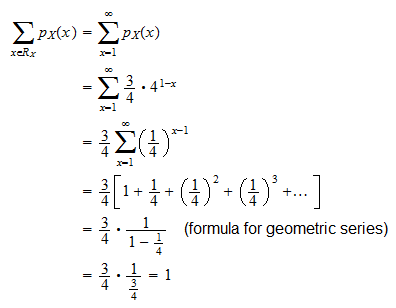StatLect

# Legitimate probability mass function

In this lecture we analyze two properties of probability mass functions (pmfs).

We prove not only that any probability mass function satisfies these two properties, but also that any function satisfying them is a legitimate pmf.

As a consequence, when we need to check whether a function is a valid pmf, we just need to verify that the two properties hold.## Properties of probability mass functions

Proposition Letbe a discrete random variable and letbe its probability mass function. Then, the functionsatisfies the following two properties:

1. Non-negativity:for any;

2. Sum over the support equals:, whereis the support of.

Proof

Remember that, by the definition of a probability mass function,is such that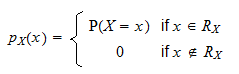Probabilities cannot be negative, thereforeand, as a consequence,. This proves Property 1 (non-negativity).

Furthermore, the probability of a sure thing must be equal to. Since, by the very definition of support, the eventis a sure thing, thenwhich proves Property 2 (sum over the support equals).

## How to verify that a pmf is valid

Any probability mass function must satisfy Properties 1 and 2 above.

By using some standard results from measure theory (omitted here), it is possible to prove that the converse is also true: any functionsatisfying the two properties above is a pmf.

Proposition Letbe a function satisfying the following two properties:

1. Non-negativity:for any;

2. Sum over the support equals:, whereis the support of.

Then, there exists a discrete random variablewhose probability mass function is.

As a consequence, we only need to check that these two properties hold when we want to prove that a function is a valid pmf.

## How to build valid pmfs

The proposition above gives us a powerful method for constructing probability mass functions.

Take a subset of the set of real numbers.

Take any functionthat is non-negative on(non-negative means thatfor any).

If the sumis well-defined and is finite and strictly positive, then defineSinceis strictly positive,is non-negative and it satisfies Property 1.

The functionalso satisfies Property 2 becauseTherefore, any functionthat is non-negative on(is chosen arbitrarily) can be used to construct a pmf if its sum overis well-defined and it is finite and strictly positive.

Example Defineand a functionas follows:Can we useto build a probability mass function? First of all, we have to check thatis non-negative. This is obviously true, becauseis always non-negative. Then, we have to check that the sum ofoverexists and is finite and strictly positive: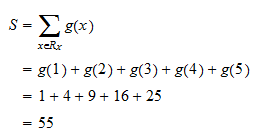Sinceexists and is finite and strictly positive, we can define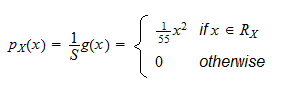By the above proposition,is a valid probability mass function.

## Solved exercises

Below you can find some exercises with explained solutions.

### Exercise 1

Consider the following function: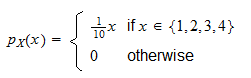Determine whetheris a valid probability mass function.

Solution

Forwe havewhile forwe haveTherefore,for anyand the non-negativity property is satisfied. The other necessary property (sum over the support equals) is also satisfied because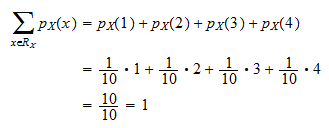### Exercise 2

Consider the following function: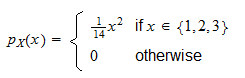Check thatis a legitimate probability mass function.

Solution

Forwe havewhile forwe haveTherefore,for anyand the non-negativity property is satisfied. The other necessary property (sum over the support equals) is also satisfied because### Exercise 3

Consider the following function: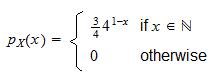Prove thatis a valid pmf.

Solution

Forwe havebecauseis strictly positive. Forwe haveTherefore,for anyand the non-negativity property is satisfied. The other necessary property (sum over the support equals) is also satisfied because